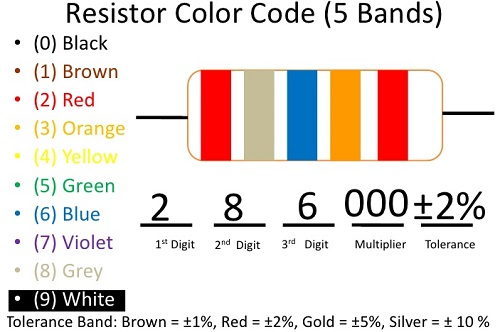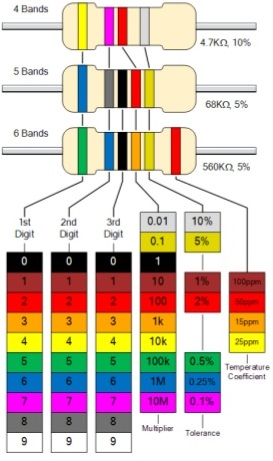# Resistor Color Code Chart & Resistor Calculator

If you want to understand resistor color coding then you are at right place where you are explained full resistor calculator depending upon the resistor colour bands which are always confusing for the electricians. There are 4, 5 and 6 bands on different resistors.

How to Read Resistor Color Code?

Resistors are color coded for easy reading. To determine or calculate the resistance and tolerance value of a given resistor color look for the gold or silver tolerance band and hold the resistor with this band to the right. Then read the resistor color code bands left to right.

Look at the 1st color band and determine its color. This may be difficult on small or oddly colored resistors. Now look at the chart given below on this page and match the “1st & 2nd resistor color code band” color to the “Digit it represents”. Write this number down.

Now look at the 2nd color band and match that color to the same resistor colour code chart. Write this number next to the 1st Digit.

Match the 3rd color band with the chart under multiplier. This number will give the number of zeros (0’s) you will multiply with the other 2 numbers by i.e. if it is 3 you will multiply with 1000 (3 zeros). Write it next to the other 2 numbers with a multiplication sign before it.

## Resistor Calculator Example

First color is red which is 2, Second color is black which is 0, Third color is yellow which is 4 so multiplier come 10,000. Tolerance band is silver which is 10% Therefore the equation is:

2 0 x 10,000 = 200,000 Ohms ± 10%Now you can easily remember the resistor colour code by remembering below given sentence.

Big Brown Rabbits Often Yield Great Big Vocal Groans When Gingerly Slapped.

### Why to use kilo Ohm & Mega Ohm in Resistance Calculator

When we calculate the resistance of a resistor we see large number of zeros, therefore prefixes are used to have a handy short name. “k” is used for kilo and means 1000 times and “M” is used for mega and means 1,000,000 times (million)

#### Another example to understand resistor calculation

We have resistor color codes Orange Orange Brown Gold resistor, lets calculate what will be resistance? The first orange means 3, The second orange means 3, The brown means 1 zero and the gold means ± 5%.

The answer is 330 ohms ± 5%

resistor-color-codes##### Standard Resistor Values

Because it is not possible to stock all possible values of resistances in a shop or component store, preferred values have been chosen to represent a usable spectrum of values for resistor color code and classes.

Depending on the accuracy required, resistances are available in 12 standard resistor values for every decade (between 1 and 10, 10 and 100, 100 and 1000 etc.) called the E12 series, 24 values for every decade, called the E24 series, and 48, 96 etc.the E12 series would cover any possible value of resistance, if the resistors have a tolerance of 10%, the E24 series 5%, and the E96 series 1%, etc.

error: Content is protected !!